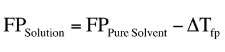Chemistry Practice Problems Freezing Point Depression Practice Problems Solution: Calculate the freezing point and boiling point of ...

🤓 Based on our data, we think this question is relevant for Professor Ivanov's class at UCD.

# Solution: Calculate the freezing point and boiling point of each aqueous solution, assuming complete dissociation of the solute.Calculate the freezing point of the solution containing 0.111 m K2S.

###### Problem

Calculate the freezing point and boiling point of each aqueous solution, assuming complete dissociation of the solute.

Calculate the freezing point of the solution containing 0.111 m K2S.

###### Solution

To calculate the freezing point of the solution we use the formula below:The pure solvent here is just water because the solution is aqueous.

Now the ∆Tfp can be calculated with the formula below:View Complete Written Solution

Freezing Point Depression

Freezing Point Depression

#### Q. Sulfuric acid in water dissociates completely into H+ and HSO4- ions. The HSO4- ion dissociates to a limited extent into H+ and SO42-. The freezing po...

Solved • Mon Dec 03 2018 16:16:53 GMT-0500 (EST)

Freezing Point Depression

#### Q. A 50.0-mL solution is initially 1.54% MgCl2 by mass and has a density of 1.05 g/mL.What is the freezing point of the solution after you add an additio...

Solved • Mon Dec 03 2018 16:16:52 GMT-0500 (EST)

Freezing Point Depression

#### Q. Calculate the freezing point and boiling point of each aqueous solution, assuming complete dissociation of the solute.a. 0.100 m K2Sb. 21.5 g of CuCl2...

Solved • Mon Dec 03 2018 16:16:46 GMT-0500 (EST)

Freezing Point Depression

#### Q. Calculate the freezing point and boiling point in each solution, assuming complete dissociation of the solute.Calculate the freezing point of a soluti...

Solved • Mon Dec 03 2018 16:16:46 GMT-0500 (EST)